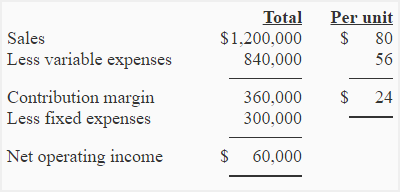# Exercise-5 (CM ratio, break-even analysis, target profit analysis, margin of safety)

Following is the contribution margin income statement of a single product company:Required:

1. Calculate break-even point in units and dollars.
2. What is the contribution margin at break-even point?
3. Compute the number of units to be sold to earn a profit of \$36,000.
4. Compute the margin of safety using original data.
5. Compute CM ratio. Compute the expected increase in monthly net operating if sales increase by \$160,000 and fixed expenses do not change.

## Solution:

### (1) Break-even point in units and dollars:

Fixed expenses/Unit contribution margin

\$300,000/\$24

12,500 units

or

(12,500 units × \$80) = \$1,000,000

### (2) Contribution margin at break-even point:

Contribution margin must be \$300,000 at break-even point because it will cover fixed costs and nothing will remain to go towards profit.

### (3) Computation of target profit:

(Fixed expenses + Target profit)/Unit contribution margin

(\$300,000 + \$36,000)/\$24

Company must sell 14,000 units of product to earn a target profit of \$36,000.

### (4) Margin of safety in dollars and percentage:

Margin of safety in dollars = Actual or budgeted sales – sales required to break-even

\$1,200,000 – \$1,000,000

\$200,000

or

Margin of safety in percentage = Margin of safety in dollars/Actual or budgeted sales

= \$200,000/\$1,200,000

=0.1667 or 16.67%

### (5) CM ratio and expected change in net operating income:

Contribution margin/Total sales

= \$360,000/\$1,200,000

= 0.30 or 30%

If the sales are increased by \$160,000 without any change in fixed expenses, the net operating income will be increased by \$48,000 as computed below:

\$160,000 × CM ratio

\$160,000 × 0.3

= \$48,000

A D V E R T I S E M E N T
2 Comments on Exercise-5 (CM ratio, break-even analysis, target profit analysis, margin of safety)
1.mitzi l. palmes

this exercise helps me in my studies.. just continue to formulate another problems with complexities for more reference. Thank you.

2.hellen

thank you very much, the exercise helps me in my studies.